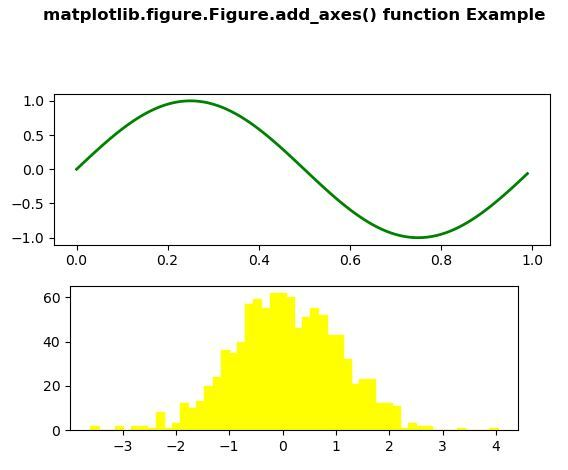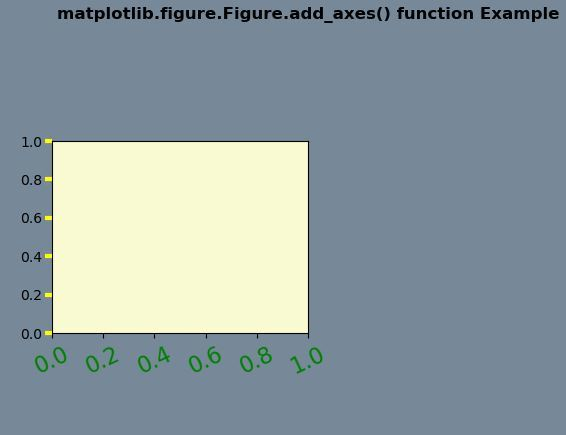Matplotlib is a library in Python and it is a numerical – mathematical extension for NumPy library. The figure module provides the top-level Artist, the Figure, which contains all the plot elements. This module is used to control the default spacing of the subplots and top-level containers for all plot elements.

The add_axes() method figure module of matplotlib library is used to add an axes to the figure.

Syntax: add_axes(self, *args, **kwargs) Parameters: This accept the following parameters that are described below:

• rect : This parameter is the dimensions [left, bottom, width, height] of the new axes.
• projection : This parameter is the projection type of the Axes.
• sharex, sharey : These parameters share the x or y axis with sharex and/or sharey.
• label : This parameter is the label for the returned axes.

Returns: This method return the axes class depends on the projection used.

Note : To understand multiple axes( multiple rectangle insertion in generated figure) easily, Think of  a rectangle which is 1 * 1 (with 0.1 as increment ).Within the rectangle we have arrange those axes with specifying ([a,b,c,d])

(a,b) is the point in southwest corner of the rectangle which we create. c represents width and d represents height of the respective rectangle.

Try this basic example on your own  to understand their placement within a rectangle.

import matplotlib.pyplot as plt
import numpy as np
figu = plt.figure()
r = figu.patch
r.set_facecolor(‘lightslategray’)

axes = figu.add_axes([0, 0.4, 0.1, 1])
axes = figu.add_axes([1, 1, 0.2, 0.3])
plt.show()

Below examples illustrate the matplotlib.figure.Figure.add_axes() function in matplotlib.figure: Example 1:

## Python3

 `# Implementation of matplotlib function` `import` `numpy as np` `import` `matplotlib.pyplot as plt`     `fig ``=` `plt.figure()` `fig.subplots_adjust(top``=``0.8``)` `ax1 ``=` `fig.add_subplot(``211``)`   `t ``=` `np.arange(``0.0``, ``1.0``, ``0.01``)` `s ``=` `np.sin(``2` `*` `np.pi ``*` `t)` `line, ``=` `ax1.plot(t, s, color``=``'green'``, lw``=``2``)`   `np.random.seed(``19680801``)`   `ax2 ``=` `fig.add_axes([``0.15``, ``0.1``, ``0.7``, ``0.3``])` `n, bins, patches ``=` `ax2.hist(np.random.randn(``1000``), ``50``,` `                            ``facecolor``=``'yellow'``,` `                            ``edgecolor``=``'yellow'``)`   `fig.suptitle('matplotlib.figure.Figure.add_axes() \` `function Example\n\n', fontweight``=``& quot` `             ``bold & quot` `             ``)`   `plt.show()`

Output:Example-2:

## Python3

 `# Implementation of matplotlib function` `import` `numpy as np` `import` `matplotlib.pyplot as plt`   `fig ``=` `plt.figure()` `rect ``=` `fig.patch` `rect.set_facecolor(``'lightslategray'``)`   `ax1 ``=` `fig.add_axes([``0.1``, ``0.3``, ``0.4``, ``0.4``])` `rect ``=` `ax1.patch` `rect.set_facecolor(``'lightgoldenrodyellow'``)`     `for` `label ``in` `ax1.xaxis.get_ticklabels():` `    ``label.set_color(``'green'``)` `    ``label.set_rotation(``25``)` `    ``label.set_fontsize(``16``)`   `for` `line ``in` `ax1.yaxis.get_ticklines():` `    ``line.set_color(``'yellow'``)` `    ``line.set_markersize(``5``)` `    ``line.set_markeredgewidth(``3``)`   `fig.suptitle('matplotlib.figure.Figure.add_axes() \` `function Example\n\n', fontweight ``=``"bold")`   `plt.show()`

Output:Whether you're preparing for your first job interview or aiming to upskill in this ever-evolving tech landscape, GeeksforGeeks Courses are your key to success. We provide top-quality content at affordable prices, all geared towards accelerating your growth in a time-bound manner. Join the millions we've already empowered, and we're here to do the same for you. Don't miss out - check it out now!

Previous
Next